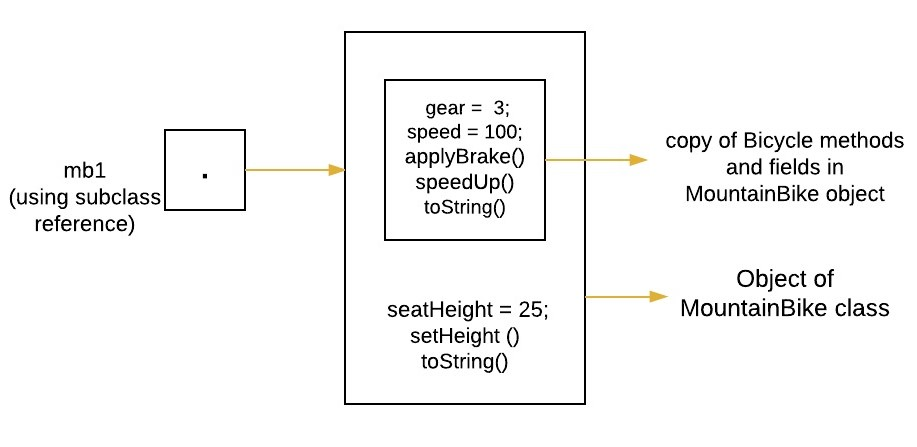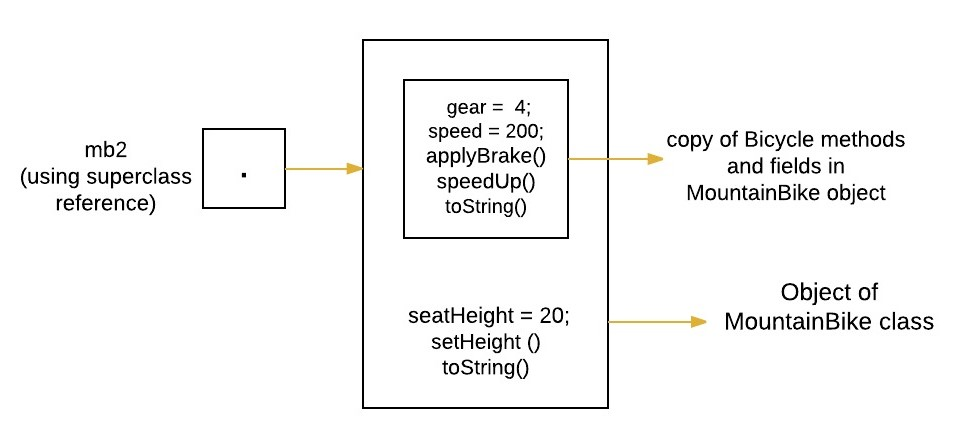# Referencing Subclass objects with Subclass vs Superclass reference

• Difficulty Level : Easy
• Last Updated : 29 Mar, 2017

Prerequisite : Inheritance
In Java, all non-static methods are based on the runtime type of the underlying object rather than the type of the reference that points to that object. Therefore, it doesn’t matter which type you use in the declaration of the object, the behavior will be the same.

How to Refer a subclass object

There are two approaches to refer a subclass object. Both have some advantages/disadvantages over the other. The declaration affect is seen on methods that are visible at compile-time.

1. First approach (Referencing using Superclass reference): A reference variable of a superclass can be used to a refer any subclass object derived from that superclass. If the methods are present in SuperClass, but overridden by SubClass, it will be the overridden method that will be executed.
2. Second approach (Referencing using subclass reference) : A subclass reference can be used to refer its object.

Consider an example explaining both the approaches.

 `// Java program to illustrate ``// referring to a subclass``// base class``class` `Bicycle ``{``    ``// the Bicycle class has two fields``    ``public` `int` `gear;``    ``public` `int` `speed;``         ` `    ``// the Bicycle class has one constructor``    ``public` `Bicycle(``int` `gear, ``int` `speed)``    ``{``        ``this``.gear = gear;``        ``this``.speed = speed;``    ``}``         ` `    ``// the Bicycle class has three methods``    ``public` `void` `applyBrake(``int` `decrement)``    ``{``        ``speed -= decrement;``    ``}``         ` `    ``public` `void` `speedUp(``int` `increment)``    ``{``        ``speed += increment;``    ``}``     ` `    ``// toString() method to print info of Bicycle``    ``public` `String toString() ``    ``{``        ``return``(``"No of gears are "``+gear``                ``+``"\n"``                ``+ ``"speed of bicycle is "``+speed);``    ``} ``}`` ` `// derived class``class` `MountainBike ``extends` `Bicycle ``{``     ` `    ``// the MountainBike subclass adds one more field``    ``public` `int` `seatHeight;`` ` `    ``// the MountainBike subclass has one constructor``    ``public` `MountainBike(``int` `gear,``int` `speed,``                        ``int` `startHeight)``    ``{``        ``// invoking base-class(Bicycle) constructor``        ``super``(gear, speed);``        ``seatHeight = startHeight;``    ``} ``         ` `    ``// the MountainBike subclass adds one more method``    ``public` `void` `setHeight(``int` `newValue)``    ``{``        ``seatHeight = newValue;``    ``} ``     ` `    ``// overriding toString() method``    ``// of Bicycle to print more info``    ``@Override``    ``public` `String toString() ``    ``{``         ` `        ``return` `(``super``.toString()+``                ``"\nseat height is "``+seatHeight);``    ``}``     ` `}`` ` `// driver class``public` `class` `Test ``{``    ``public` `static` `void` `main(String args[]) ``    ``{``        ``// using superclass reference``        ``// first approach``        ``Bicycle mb2 = ``new` `MountainBike(``4``, ``200``, ``20``);``         ` `        ``// using subclass reference( )``        ``// second approach``        ``MountainBike mb1 = ``new` `MountainBike(``3``, ``100``, ``25``);``         ` `        ``System.out.println(``"seat height of first bicycle is "` `                                            ``+ mb1.seatHeight);``             ` `        ``// In case of overridden methods``        ``// always subclass ``        ``// method will be executed``        ``System.out.println(mb1.toString());``        ``System.out.println(mb2.toString());`` ` `        ``/* The following statement is invalid because Bicycle``        ``does not define a seatHeight. ``        ``// System.out.println("seat height of second bicycle is " ``                                                ``+ mb2.seatHeight); */``                     ` `        ``/* The following statement is invalid because Bicycle``        ``does not define setHeight() method. ``        ``mb2.setHeight(21);*/`` ` `    ``}``}`

Output:

```seat height of first bicycle is 25
No of gears are 3
speed of bicycle is 100
seat height is 25
No of gears are 4
speed of bicycle is 200
seat height is 20
```

Explanation of above program :

• The object of MountainBike class is created which is referred by using subclass reference ‘mb1’. Using this reference we will have access both parts(methods and variables) of the object defined by the superclass or subclass. See below image for clear understanding.
```MountainBike mb1 = new MountainBike(3, 100, 25);
```• Now we again create object of MountainBike class but this time it is referred by using superclass Bicycle reference ‘mb2’. Using this reference we will have access only to those parts(methods and variables) of the object defined by the superclass.

```Bicycle mb2 = new MountainBike(4, 200, 20);
```• Since the reference ‘mb1’ have access to field ‘seatHeight’, so we print this on console.
```System.out.println("seat height of first bicycle  is " + mb1.seatHeight);
```
• If there are methods present in super class, but overridden by subclass, and if object of subclass is created, then whatever reference we use(either subclass or superclass), it will always be the overridden method in subclass that will be executed. So below two statements will call toString() method of MountainBike class.

```System.out.println(mb1.toString());
System.out.println(mb2.toString());
```
• Since the reference made by ‘mb2’ is of type Bicycle , so we will get compile time error in below statement.

```System.out.println("seat height of second bicycle  is " + mb2.seatHeight);
```
• Again the reference made by ‘mb2’ is of type Bicycle , so we will get compile time error in below statement.
```mb2.setHeight(21);
```

Use of type casting

In above example, we have seen that by using reference ‘mb2’ of type Bicycle, we are unable to call subclass specific methods or access subclass fields. This problem can be solved using type casting in java. For example, we can declare another reference say ‘mb3’ of type MountainBike and assign it to ‘mb2’ using typecasting.

```// declaring MountainBike reference
MountainBike mb3;

// assigning mb3 to mb2 using typecasting.
mb3 = (MountainBike)mb2;
```

So, now the following statements are valid.

```System.out.println("seat height of second bicycle  is " + mb3.seatHeight);
mb3.setHeight(21);
```

When to go for first approach (Referencing using superclass reference)

If we don’t know exact runtime type of an object, then we should use this approach. For example, consider an ArrayList containing different objects at different indices. Now when we try to get elements of arraylist using ArrayList.get(int index) method then we must use Object reference, as in this case, we don’t know exact runtime type of an object. For example :

 `/* Java program to illustrate referring to a subclass``using superclass reference variable */``import` `java.util.ArrayList;`` ` `public` `class` `Test ``{``       ``public` `static` `void` `main(String args[]) ``       ``{``           ``ArrayList al = ``new` `ArrayList(``2``);``            ` `           ``// adding String object to al``           ``al.add(``new` `String(``"GeeksForGeeks"``));``            ` `           ``// adding Integer object to al``           ``al.add(``new` `Integer(``5``));``            ` `           ``// getting all elements using Object reference``           ``for` `(Object object : al)``           ``{``               ``System.out.println(object);``           ``}    ``       ``}``}`

Output:

```GeeksForGeeks
5
```

Advantage : We can use superclass reference to hold any subclass object derived from it.

Disadvantage : By using superclass reference, we will have access only to those parts(methods and variables) of the object defined by the superclass. For example, we can not access seatHeight variable or call setHeight(int newValue) method using Bicycle reference in above first example. This is because they are defined in subclass not in the superclass.

When to go for second approach (Referencing using subclass reference)

If we know the exact runtime type of an object, then this approach is better. Using this approach, we can also call a particular object specific methods. For example :

 `/* Java program to illustrate referring to a subclass``using subclass reference variable */``import` `java.util.ArrayList;`` ` `public` `class` `Test ``{``       ``public` `static` `void` `main(String args[]) ``       ``{``           ``ArrayList al = ``new` `ArrayList(``2``);``            ` `           ``// adding String objects to al``           ``al.add(``new` `String(``"GeeksForGeeks"``));``           ``al.add(``new` `String(``"for java archives"``));``            ` `             ` `           ``// getting  elements using String reference``            ``String str1 = (String)al.get(``0``);``            ``String str2 = (String)al.get(``1``);``             ` `            ``System.out.println(str1);``            ``System.out.println(str2);``             ` `            ``// using String class specific method``            ``System.out.println(str1.length());``            ``System.out.println(str2.substring(``4``,``8``));``       ``}``}`

Output:

```GeeksForGeeks
for java archives
13
java
```

Advantage : By using subclass reference, we will have access to both parts(methods and variables) of the object defined by the superclass or subclass. For example, we can call setHeight(int newValue) method or speedUp(int increment) method using MountainBike reference in above first example.

Disadvantage : We can use subclass reference to hold only for that particular subclass objects only.

Reference: StackOverflow

This article is contributed by Gaurav Miglani. If you like GeeksforGeeks and would like to contribute, you can also write an article using contribute.geeksforgeeks.org or mail your article to contribute@geeksforgeeks.org. See your article appearing on the GeeksforGeeks main page and help other Geeks.